# Python, MATLAB, Julia, R code: Chapter 1

Acknowledgement: The Julia code is written by the contributors listed here.

### Chapter 1.1

#### Visualizing a geometric series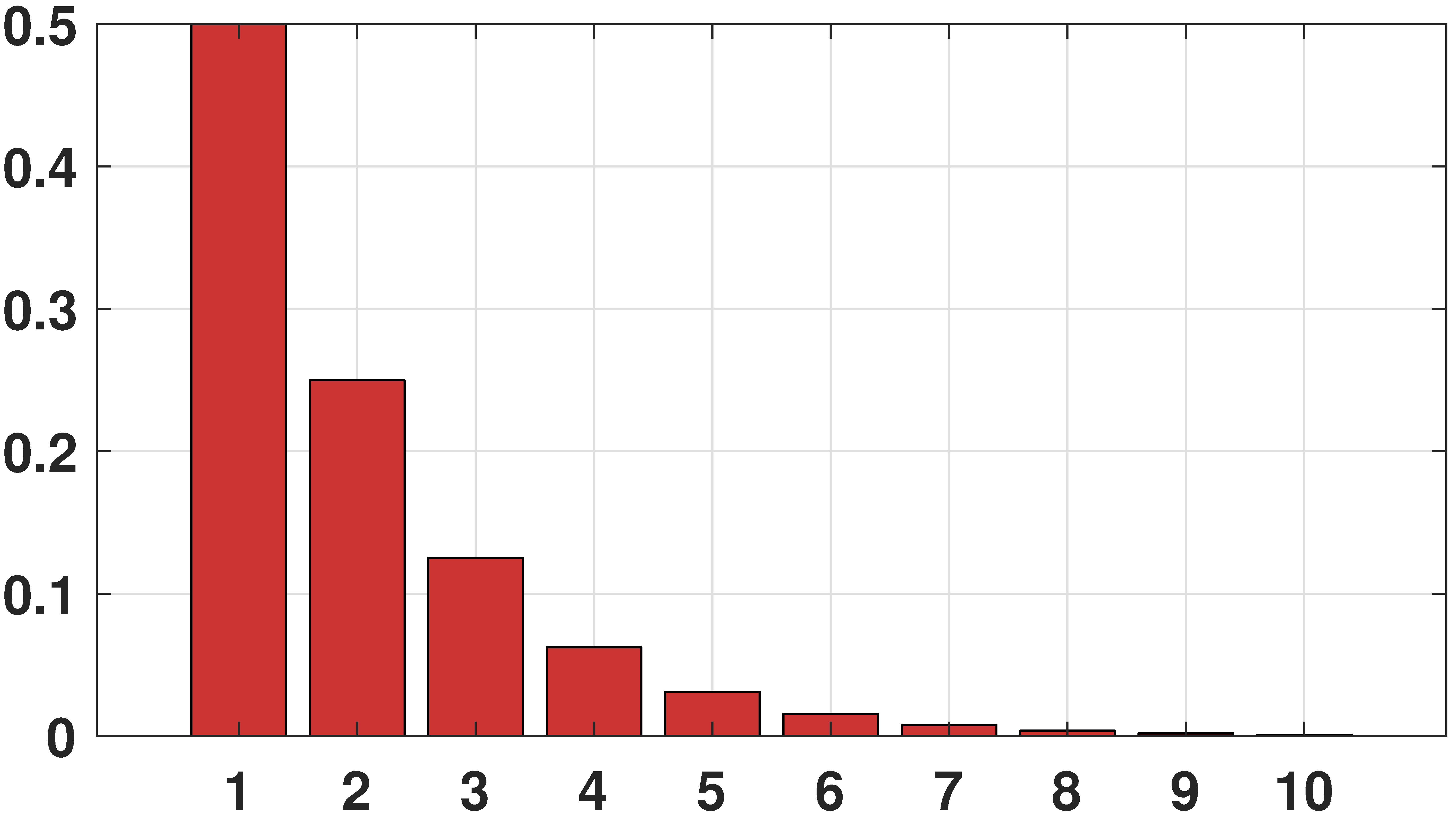```# MATLAB code to generate a geometric sequence
p = 1/2;
n = 1:10;
X = p.^n;
bar(n,X,'FaceColor',[0.8, 0.2,0.2]);
```
```# Python code to generate a geometric sequence
import numpy as np
import matplotlib.pyplot as plt
p = 1/2
n = np.arange(0,10)
X = np.power(p,n)
plt.bar(n,X)
```
```# Julia code to generate a geometric series
using StatsPlots:bar
p = 0.5
n = 1:10
X = p .^ n
bar(n, X, legend=false)
```
```# R code to generate a geometric series
p <- 1/2
n <- seq(0, 9)
X <- `^`(p,n)
barplot(X)
```

#### Compute N choose K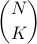```# MATLAB code to compute (N choose K) and K!
n = 10;
k = 2;
nchoosek(n,k)
factorial(k)
```
```# Python code to compute (N choose K) and K!
from scipy.special import comb, factorial
n = 10
k = 2
comb(n, k)
factorial(k)
```
```# Julia code to compute (N choose K) and K!
n = 10
k = 2
binomial(n, k)
factorial(k)
```
```# R code to compute (N choose K) and K!
n <- 10
k <- 2
choose(n,k)
factorial(k)
```

### Chapter 1.4

#### Inner product of two vectors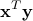```# MATLAB code to perform an inner product
x = [1 0 -1];
y = [3 2 0];
z = x'*y;
```
```# Python code to perform an inner product
import numpy as np
x = np.array([,,[-1]])
y = np.array([,,])
z = np.dot(np.transpose(x),y)
print(z)
```
```# Julia code to perform an inner product
x = [1, 0, -1]
y = [3, 2, 0]
x'y
```
```# R code to perform an inner product
x <- c(1,0,-1)
y <- c(3,2,0)
z <- t(x) #*# y
print(z)
```

#### Norm of a vector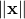```# MATLAB code to compute the norm
x = [1 0 -1];
x_norm = norm(x);
```
```# Python code to compute the norm
import numpy as np
x = np.array([,,[-1]])
x_norm = np.linalg.norm(x)
```
```# Julia code to compute the norm
using LinearAlgebra:norm
x = [1, 0, -1]
norm(x)
```
```# R code to compute the norm
x <- c(1,0,-1)
x_norm <- norm(x, type = "2")
# "2" specifies the “spectral” or 2-norm, which is the largest singular value of x
print(x_norm)
```

#### Weighted norm of a vector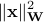```# MATLAB code to compute the weighted norm
W = [1 2 3; 4 5 6; 7 8 9];
x = [2; -1; 1];
z = x'*W*x
```
```# Python code to compute the weighted norm
import numpy as np
W = np.array([[1, 2, 3], [4, 5, 6], [7, 8, 9]])
x = np.array([,[-1],])
z = np.dot(x.T, np.dot(W,x))
print(z)
```
```# Julia code to compute the weighted norm
W = [1. 2. 3.; 4. 5. 6.; 7. 8. 9.]
x = [2, -1, 1]
z = x'W*x
```
```# R code to compute the weighted norm
W <- matrix(1:9, ncol = 3, nrow = 3)
x <- matrix(c(2,-1,1))
z <- t(x) #*# (W #*# x)
print(z)
```

#### Matrix inverse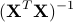```# Python code to compute a matrix inverse
import numpy as np
X      = np.array([[1, 3], [-2, 7], [0, 1]])
XtX    = np.dot(X.T, X)
XtXinv = np.linalg.inv(XtX)
print(XtXinv)
```
```# R code to compute a matrix inverse
X <- matrix(c(1,-2,0,3,7,1), nrow = 3, ncol = 2)
XtX <- t(X) #*# X
Xtxinv <- solve(XtX)
print(Xtxinv)
```

#### System of linear equation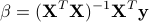```# MATLAB code to solve X beta = y
X      = [1 3; -2 7; 0 1];
y      = [2; 1; 0];
beta   = X\y;
```
```# Python code to solve X beta = y
import numpy as np
X      = np.array([[1, 3], [-2, 7], [0, 1]])
y      = np.array([,,])
beta   = np.linalg.lstsq(X, y, rcond=None)
print(beta)
```
```# Julia code to solve X beta = y
X = [1. 3.; -2. 7.; 0. 1.]
y = [2., 1., 0.]
beta = X\y
```
```# R code to solve X beta = y
X <- matrix(c(1,-2,0,3,7,1), nrow = 3, ncol = 2)
y <- matrix(c(2,1,0))
beta <- solve(qr(X), y)
print(beta)
```